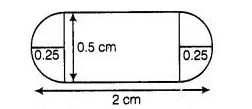# A medicine-capsule is in the shape of a cylinder

Question:

A medicine-capsule is in the shape of a cylinder of diameter 0.5 cm with two hemispheres stuck to each of its ends. The length of entire capsule is 2

cm. The capacity of the capsule is

(a) 0.36 cm3

(b) 0.35 cm3

(c) 0.34 cm3

(d) 0.33 cm3

Solution:

(a) Given, diameter of cylinder = Diameter of hemisphere = 0.5 cm

[since, both hemispheres are attach with cylinder]

$\therefore$ Radius of cylinder $(r)=$ radius of hemisphere $(r)=\frac{0.5}{2}=0.25 \mathrm{~cm}$ $[\because$ diameter $=2 \times$ radius $]$and total length of capsule $=2 \mathrm{~cm}$

$\therefore$ Length of cylindrical part of capsule,

$h=$ Lenath of capsule - Radius of both hemispheres

$=2-(0.25+0.25)=1.5 \mathrm{~cm}$

Now canacity of cansule $=$ Volume of cylindrical part $+2 \times$ Volume of hemisphere

$=\pi r^{2} h+2 \times \frac{2}{3} \pi r^{3}$

$\left[\because\right.$ volume of cylinder $=\pi \times(\text { radius })^{2} \times$ height and volume of hemispere $\left.=\frac{2}{3} \pi(\text { radius })^{3}\right]$

$=\frac{22}{7}\left[(0.25)^{2} \times 1.5+\frac{4}{3} \times(0.25)^{3}\right]$

$=\frac{22}{7}[0.09375+0.0208]$

$=\frac{22}{7} \times 0.11455=0.36 \mathrm{~cm}^{3}$

Hence, the capacity of capsule is $0.36 \mathrm{~cm}^{3}$